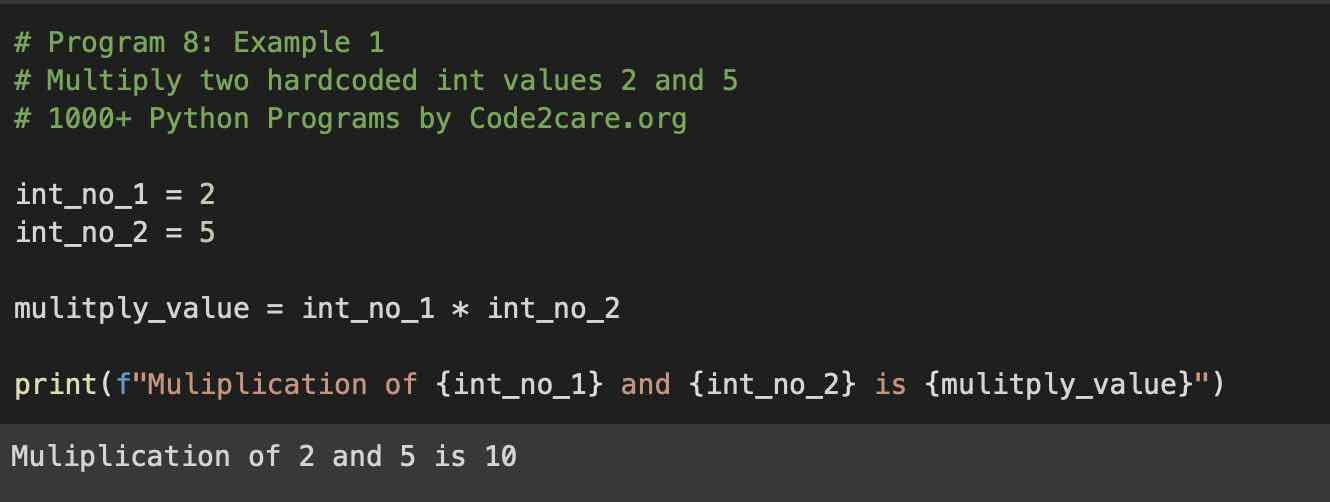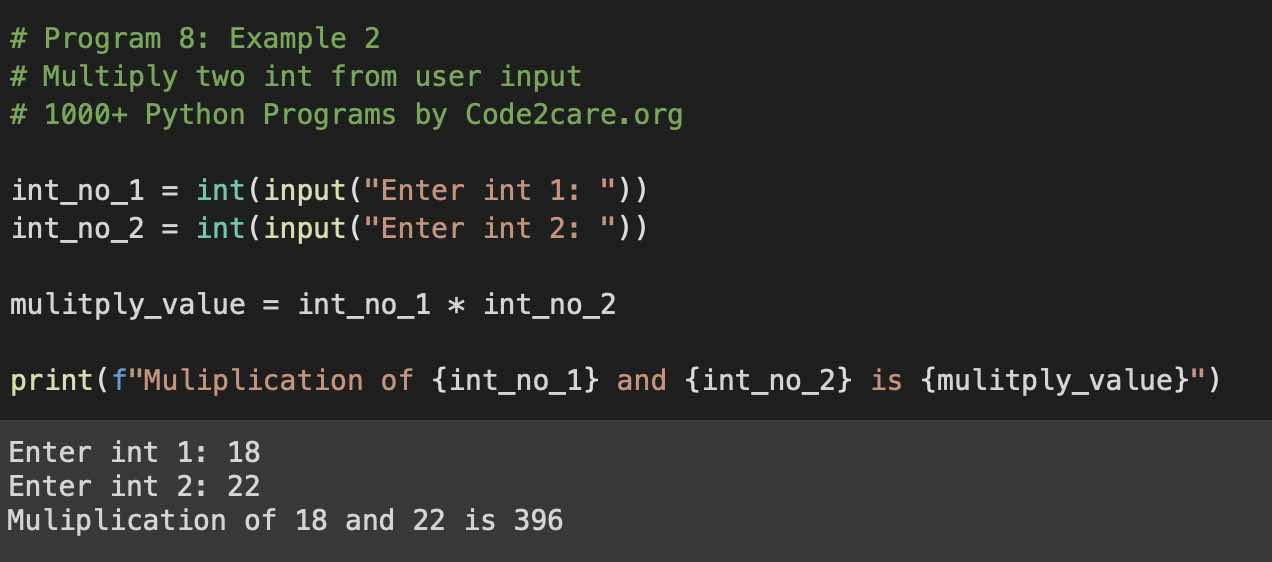# Program 8: Multiply Two Numbers - 1000+ Python Programs

Question 8)

Write a program in Python to multiply two numbers.
Write a program in Python to multiply numbers 2 and 5 numbers.
Write a program to multiply two numbers by taking user input using Python Programming.
Program to find the multiply two integer values in Python.
Program to find the multiply two float values in Python.

## Code Example 1: Multiply two Integers

``````# Program 8: Example 1
# Multiply two hardcoded int values 2 and 5
# 1000+ Python Programs by Code2care.org

int_no_1 = 2
int_no_2 = 2

mulitply_value = int_no_1 * int_no_2

print(f"Multiplication of {int_no_1} and {int_no_2} is {mulitply_value}")``````
Output:

Multiplication of 2 and 5 is 10## Code Example 2: Multiply two integer numbers from user input

``````# Program 8: Example 2
# Multiply two ints from the user input
# 1000+ Python Programs by Code2care.org

int_no_1 = int(input("Enter int 1: "))
int_no_2 = int(input("Enter int 2: "))

mulitply_value = int_no_1 * int_no_2

print(f"Multiplication of {int_no_1} and {int_no_2} is {mulitply_value}")``````
Output:

Enter int 1: 18
Enter int 2: 22
Multiplication of 18 and 22 is 396## Code Example 3: Multiply two floats numbers from user input

``````# floats 8: Example 3
# Multiply two ints from the user input
# 1000+ Python Programs by Code2care.org

float_no_1 = float(input("Enter int 1: "))
float_no_2 = float(input("Enter int 2: "))

mulitply_value = float_no_1 * float_no_2

print(f"Multiplication of {float_no_1} and {float_no_2} is {mulitply_value}")``````
Output:

Enter int 1: 10.4
Enter int 2: 2.4
Multiplication of 10.4 and 2.4 is 24.96

## Explaination:

List of functions used,

1. print(): Built-in function to print a string on the console with f-strings formatting.
2. float(): Built-in function to convert String argument to float value.
3. input(): Built-in function to accept user input

### Steps:

1. Create a variable to hold the first integer/float
2. Create a variable to hold a second integer/float
3. Create a third variable to hold the multiplication of two variables using the * (asterisk) multiplication operator.
4. Print the result using print() function.